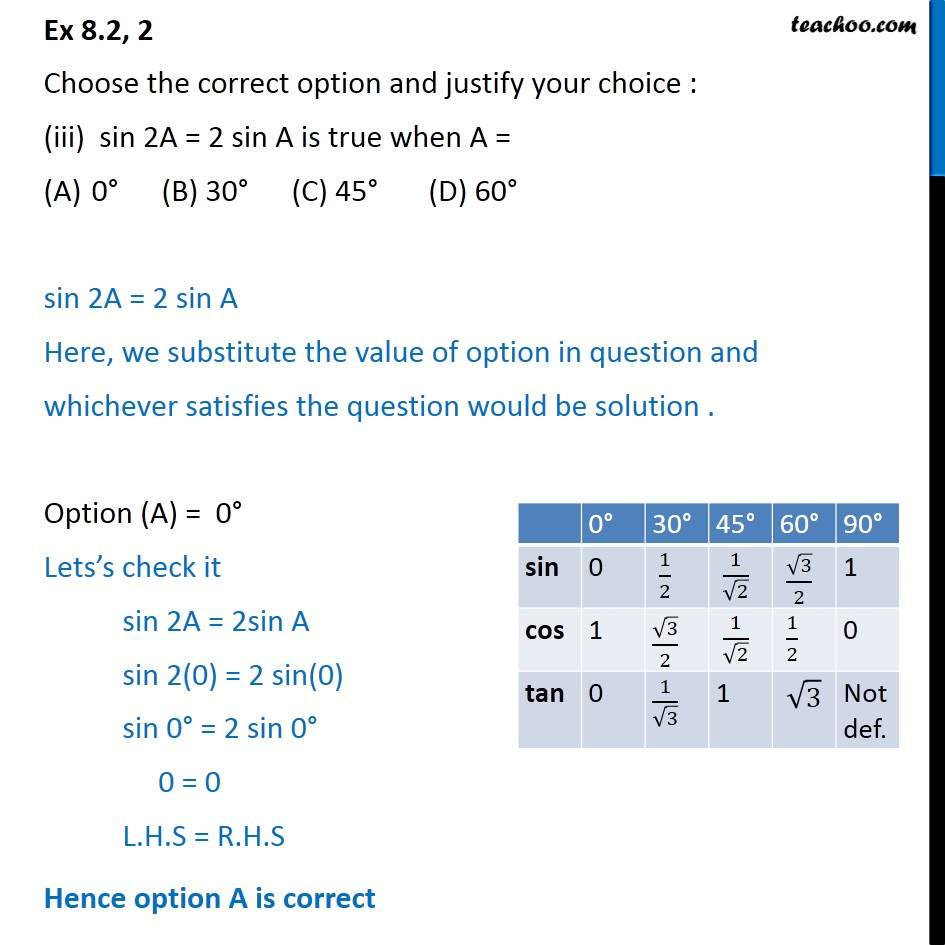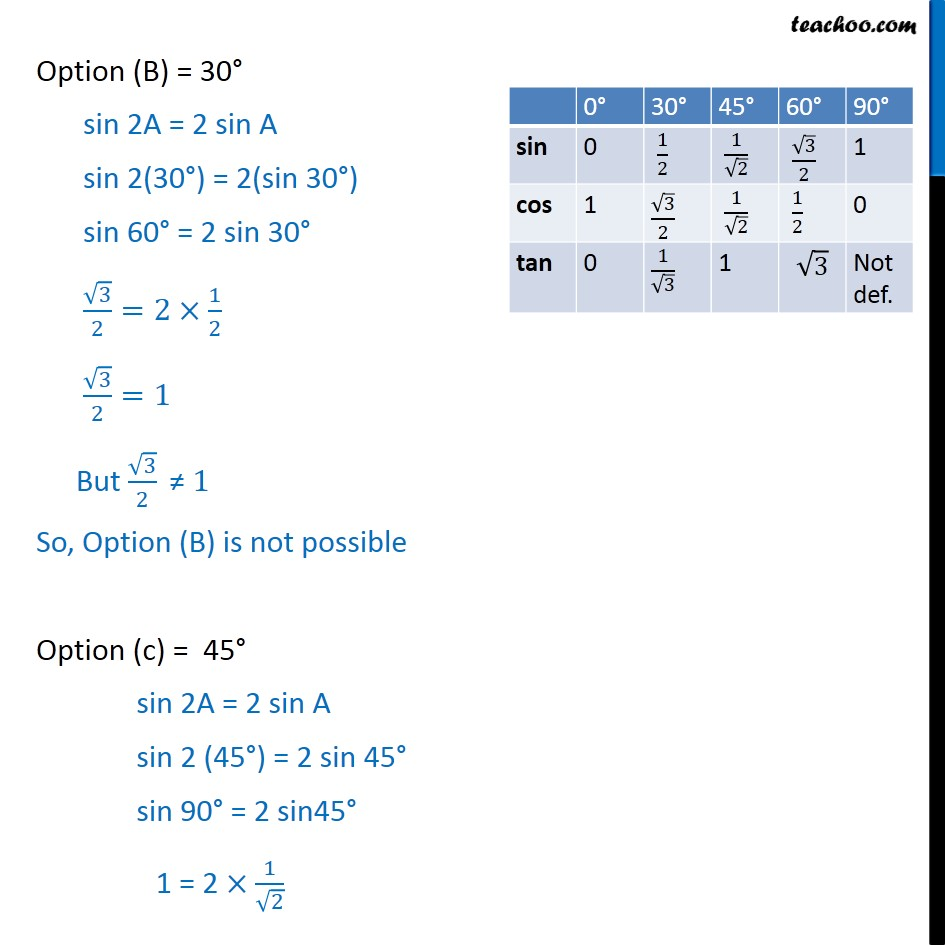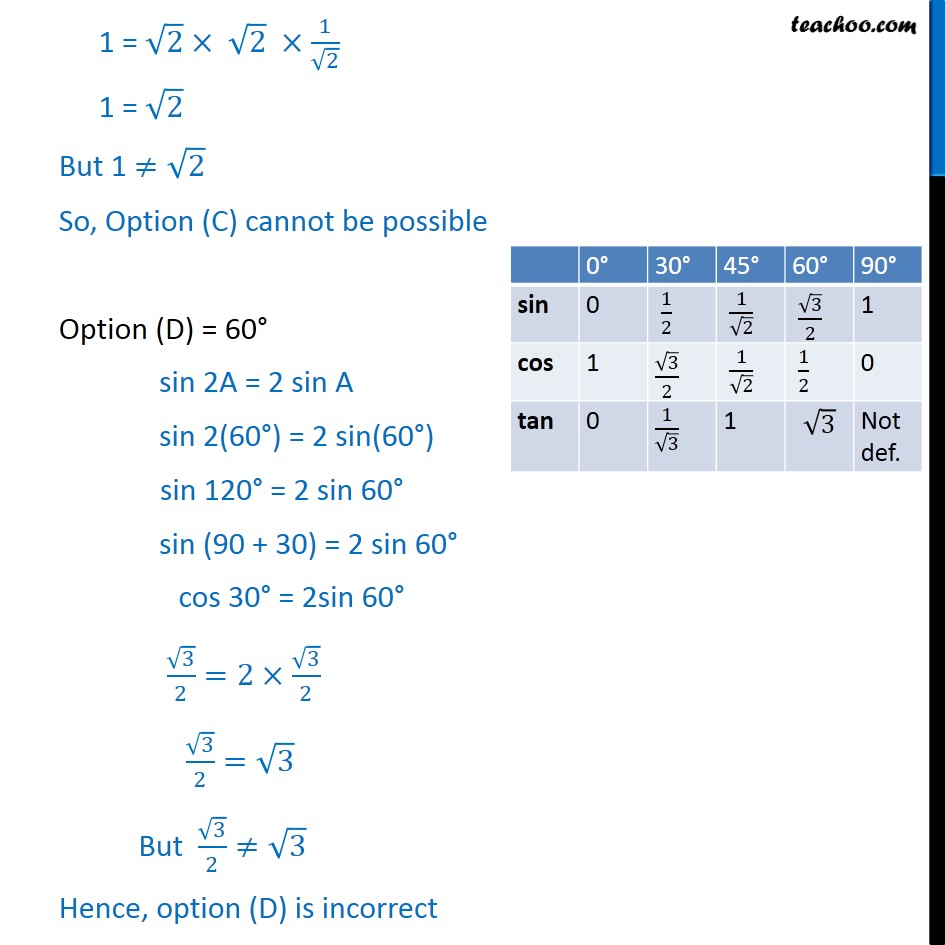1. Chapter 8 Class 10 Introduction to Trignometry (Term 1)
2. Serial order wise
3. Ex 8.2

Transcript

Ex 8.2, 2 Choose the correct option and justify your choice : (iii) sin 2A = 2 sin A is true when A = 0 (B) 30 (C) 45 (D) 60 sin 2A = 2 sin A Here, we substitute the value of option in question and whichever satisfies the question would be solution . Option (A) = 0 Lets s check it sin 2A = 2sin A sin 2(0) = 2 sin(0) sin 0 = 2 sin 0 0 = 0 L.H.S = R.H.S Hence option A is correct Option (B) = 30 sin 2A = 2 sin A sin 2(30 ) = 2(sin 30 ) sin 60 = 2 sin 30 3/2=2 1/2 3/2=1 But 3/2 " " 1 So, Option (B) is not possible Option (c) = 45 sin 2A = 2 sin A sin 2 (45 ) = 2 sin 45 sin 90 = 2 sin45 1 = 2 1/ 2 1 = 2 2 1/ 2 1 = 2 But 1 2 So, Option (C) cannot be possible Option (D) = 60 sin 2A = 2 sin A sin 2(60 ) = 2 sin(60 ) sin 120 = 2 sin 60 sin (90 + 30) = 2 sin 60 cos 30 = 2sin 60 3/2=2 3/2 3/2= 3 But 3/2 3 Hence, option (D) is incorrect So, only option (A) is correct